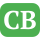CppBuzz.com

# C++ program to create new user defined data type using structure

#include<iostream> using namespace std; //We are creating new data type 'student' using structure. typedef struct{ char sex; //eith M or F int age; // +ve value int std; // 1 to 12 int rollNo; // +ve number char fname; char lname; }student; //student is new data type int main() { //declaring array of new data type 'student' student obj; for(int i = 0; i<3; i++) { cout<<"\n Enter First Name of student : "; cin>>obj[i].fname; cout<<"\n Enter Last Name of student : "; cin>>obj[i].lname; cout<<"\n Enter sex : "; cin>>obj[i].sex; cout<<"\n Enter Age : "; cin>>obj[i].age; cout<<"\n Enter std (class) : "; cin>>obj[i].std; cout<<"\n Enter Roll No : "; cin>>obj[i].rollNo; } for(int i = 0; i<3; i++) { cout<<"\n\n Name of student : "<< obj[i].fname <<" "<<obj[i].lname; cout<<"\t Sex : "<< obj[i].sex; cout<<"\t Age : "<<obj[i].age; cout<<"\t Std (class) : "<< obj[i].std; cout<<"\t Roll No : "<<obj[i].rollNo; } return 0; }